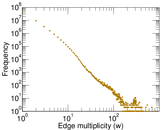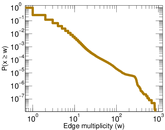# Reuters

This is the bipartite network of story–word inclusions in documents that appeared in Reuters news stories collected in the Reuters Corpus, Volume 1 (RCV1). Left nodes represent stories; right nodes represent words. An edge represents a story–word inclusion.

 Code `RE` Internal name `reuters` Name Reuters Data source http://trec.nist.gov/data/reuters/reuters.html AvailabilityDataset is available for download Consistency checkDataset passed all tests Category Text network Node meaning Story, word Edge meaning Inclusion Network formatBipartite, undirected Edge typeUnweighted, multiple edges

## Statistics

 Size n = 1,065,176 Left size n1 = 781,265 Right size n2 = 283,911 Volume m = 96,903,520 Unique edge count m̿ = 60,569,726 Wedge count s = 1,546,388,153,215 Claw count z = 64,992,574,078,209,184 Cross count x = 2.950 23 × 1021 Maximum degree dmax = 345,056 Maximum left degree d1max = 1,585 Maximum right degree d2max = 345,056 Average degree d = 181.948 Average left degree d1 = 124.034 Average right degree d2 = 341.317 Fill p = 0.000 273 071 Average edge multiplicity m̃ = 1.599 87 Size of LCC N = 1,065,175 Diameter δ = 6 50-Percentile effective diameter δ0.5 = 2.097 31 90-Percentile effective diameter δ0.9 = 3.331 29 Median distance δM = 3 Mean distance δm = 2.693 82 Gini coefficient G = 0.680 191 Balanced inequality ratio P = 0.247 840 Left balanced inequality ratio P1 = 0.345 764 Right balanced inequality ratio P2 = 0.042 455 4 Relative edge distribution entropy Her = 0.826 289 Power law exponent γ = 1.295 75 Tail power law exponent γt = 2.511 00 Degree assortativity ρ = −0.124 689 Degree assortativity p-value pρ = 0.000 00 Spectral norm α = 6,502.10 Spectral separation |λ1[A] / λ2[A]| = 1.350 62

## Plots

### Degree distribution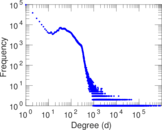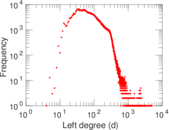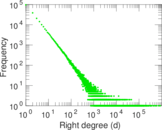### Cumulative degree distribution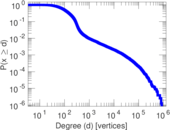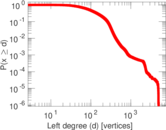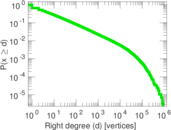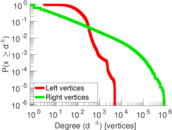### Lorenz curve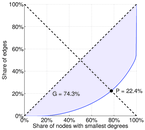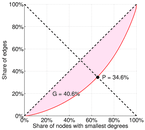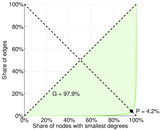### Spectral distribution of the adjacency matrix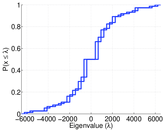### Spectral distribution of the normalized adjacency matrix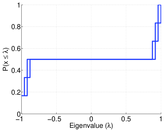### Spectral distribution of the Laplacian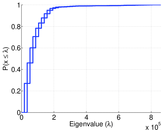### Spectral graph drawing based on the adjacency matrix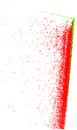### Spectral graph drawing based on the normalized adjacency matrix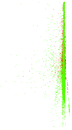### Hop distribution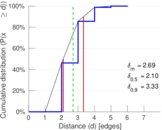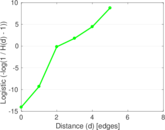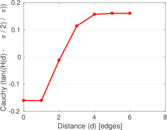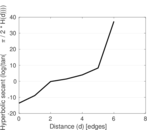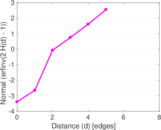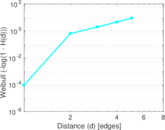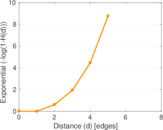### Edge weight/multiplicity distribution